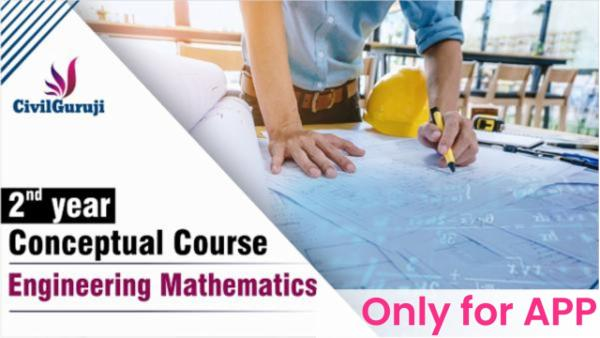# Engineering Mathematics

starstarstarstarstar 5.0 (ratings)

Language: Hindi

Why this course?

# Course Curriculum

 DEMO L01 Introduction of Engineering Mathematics (5:00) L02 Introduction of Integral Calculus (8:00) L01 Introduction of Engineering Mathematics (5:00) L02 Introduction of Integral Calculus L03 Introduction of differentiation calculus (6:00) L04 Introduction of vector calculus (5:00) L05 Introduction of probability (7:00) L06 Real life experience of differential equation (5:00) L07 Introduction of Fourier transform L08 Divergence & curl (7:00) L09 Maxima & minima of two variables (6:00) L10 Gradient & directional derivative (4:00) L11 Integration basic Formula's (6:00) L12 Introduction of Laplace Transform & basic formula (7:00) L13 Partial differentiation and it application

# Reviews

5
star star star star star
people 4 total
 5 4 4 0 3 0 2 0 1 0

# How to Use

After successful purchase, this item would be added to your courses.You can access your courses in the following ways :

• From the computer, you can access your courses after successful login
• For other devices, you can access your library using this web app through browser of your device.